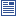Accessibility options:

# Functions and Graphs resources

Show me all resources applicable to

### Community Project (1)Exponential and Logarithm for Economics and Business Studies (SOURCE)
Latex source, image files and metadata for the Fact & Formulae leaflet "Exponential and Logarithm for Economics and Business Studies" contributed to the mathcentre Community Project by Morgiane Richard (University of Aberdeen) and reviewed by Shazia Ahmed (University of Glasgow) and Anthony Cronin (University College Dublin).

### Facts & Formulae Leaflets (1)Exponential and Logarithm for Economics and Business Studies
Overview of the properties of the functions e and ln and their applications in Economics. This leaflet has been contributed to the mathcentre Community Project by Morgiane Richard (University of Aberdeen) and reviewed by Shazia Ahmed (University of Glasgow) and Anthony Cronin (University College Dublin).

### Quick Reference (5)Linear relationships
In many business applications, two quantities are related linearly. This means a graph of their relationship forms a straight line. This leaflet discusses one form of the mathematical equation which describes linear relationships.The exponential constant e
The letter e is used in many mathematical calculations to stand for a particular number known as the exponential constant. This leaflet provides information about this important constant, and the related exponential function.The graph of a function
A very useful pictorial representation of a function is the graph. In this leaflet, we remind you of important conventions when plotting a graph.The slope-intercept form
This leaflet explains the slope-intercept form of an equation describing a straight-line.What is a function?
A quantity whose value can change is known as a variable. Functions are used to describe the rules which define the ways in which such a change can occur. The purpose of this leaflet is to explain functions and their notation.